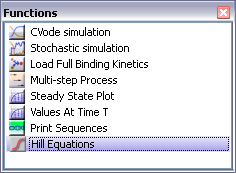## Friday, January 16, 2009

### Genetic Networks and Hill Equations

The new AutoRegulatoryNetwork plugin keeps track of how the genetic parts, such as promoters, coding sequence, etc. are moved. Whenever coding parts are placed downstream of a promoter, the coding parts will receive an Assignment rule that sets the coding part's value equal to the promoter part's value. Remember that value refers to the the activity numerical attribute for genetic parts such as promoter, rbs, coding, etc.

The AutoRegulatoryNetwork also provides actions in the context menu (mouse right click) that allow users to automatically place degradation reactions on species or transcription factor binding connections on regulatory parts.

The AutoRegulatoryNetwork is a nice combination with the Hill Equations python function, which automatically generates the Assignment rule for promoters. Select a promoter with one or more transcription factors bound to it, and click the Hill equation button.The Hill Equations function is written in Python. Below is the code:

import pytc
items = pytc.selectedItems();
promoters = [];
pytc.errorReport("",0);
for i in items:
if pytc.isA(i,"Regulatory"):
promoters.append(i);

if (len(promoters) > 0):
k = pytc.getFromList("Select the logical function to approximate:",["AND","OR","NOT","XOR"]);
for i in promoters:
fracs = [];
indiv = [];
connectors = pytc.getConnectionsIn(i);
for c in connectors:
if pytc.isA(c,"Transcription Regulation"):
cname = pytc.getName(c);
parts = pytc.getConnectedPartsIn(c);
pnames = pytc.getNames(parts);
for n in pnames:
s = "(" + n + "/" + cname + ".kd)";
indiv.append(s);
s = "(1+pow(" + s + "," + cname + ".h))";
fracs.append(s);

rate = "";
if k == 0:
rate = " * ".join(indiv) + "/(1.0 + " + "*".join(fracs) + ")";
elif k == 1:
rate = "(" + " * ".join(fracs) + ")/(1.0 + " + "*".join(fracs) + ")";
elif k == 2:
rate = " 1.0 /(1.0 + " + "*".join(fracs) + ")";
elif k == 3:
rate = "(" + " + ".join(indiv) + ")/(1 + " + "*".join(fracs) + ")";
pytc.write(rate+"\n");
if (len(rate) > 0):
pytc.setTextData(i,"Assignments",pytc.getName(i),"function",rate);
else:
pytc.errorReport("no regulatory elements selected\n",0);

### Events and Assignment FunctionsEvents allow you to define a condition and an event that will occur under those conditions. For example, IF B <= 1 THEN a =10 will set the value of A to 10 whenever B's value becomes less that 1.

An assignment rule lets you define a function, such as a A.f = 1 + sin(time) * B, or something else like that. You can then use the name A.f in other parts of the model.
Important: you can specify that A = 1 + sim(time).  This will make A itself into a boundary variable rather than a regular variable.